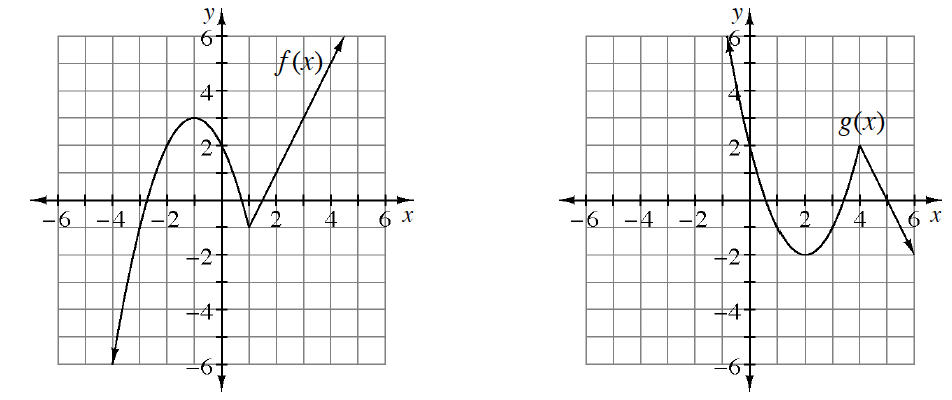### Home > PC3 > Chapter 8 > Lesson 8.2.1 > Problem8-64

8-64.

The graphs of $y=f(x)$ and $y=g(x)$ are shown below. Write an equation in terms of $f(x)$ that will transform the graph of $y = f(x)$ into $y = g(x)$. Homework Help ✎$g(x)$ is a vertical reflection of $f(x)$.

The function is then shifted to the right $3$ units.

The function is then shifted up $1$ unit.